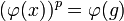Central implies normal satisfying the subgroup-to-quotient powering-invariance implication

This article gives the statement and possibly, proof, of an implication relation between two subgroup properties. That is, it states that every subgroup satisfying the first subgroup property (i.e., central subgroup) must also satisfy the second subgroup property (i.e., normal subgroup satisfying the subgroup-to-quotient powering-invariance implication)
View all subgroup property implications | View all subgroup property non-implications
Get more facts about central subgroup|Get more facts about normal subgroup satisfying the subgroup-to-quotient powering-invariance implication

Statement

Original formulation

Suppose$G$ is a group and$H$ is a central subgroup of$G$. Note that central implies normal, so$H$ is normal. Then,$H$ is a normal subgroup satisfying the subgroup-to-quotient powering-invariance implication in$G$: if$p$ is a prime number such that both$G$ and$H$ are$p$-powered, so is the quotient group$G/H$.

Corollary formulation

Suppose$G$ is a group and$H$ is a subgroup of$G$ satisfying the following two conditions:

1.$H$ is a central subgroup of$G$, i.e., every element of$H$ commutes with every element of$G$.
2.$H$ is a powering-invariant subgroup of$G$, i.e., for any prime$p$ such that$G$ is powered over$p$, so is$H$.

Then,$H$ is a quotient-powering-invariant subgroup of$G$, i.e.,for any prime$p$ such that$G$ is powered over$p$, so is the quotient group$G/H$.

Proof

Proof for original formulation

This proof uses a tabular format for presentation. Provide feedback on tabular proof formats in a survey (opens in new window/tab) | Learn more about tabular proof formats|View all pages on facts with proofs in tabular format

Given: A group$G$. A central subgroup$H$ of$G$A prime number$p$ such that$G$ is powered over$p$, i.e., every element of$G$ has a unique$p^{th}$ root in$G$.$H$ is also powered over$p$. Let$\varphi:G \to G/H$ be the quotient map. An element$a \in G/H$.

To prove: There is a unique element$b \in G/H$ satisfying$b^p = a$.

Proof:

Step no. Assertion/construction Facts used Given data used Previous steps used Explanation
1 Let$g \in G$ be such that$\varphi(g) = a$.$\varphi:G \to G/H$ is surjective by definition.
2 There exists$x \in G$ such that$x^p = g$. Remember this$x$.$G$ is powered over$p$ (we only use the divisibility aspect here, not the uniqueness). direct.
3 For any$u \in H$, there exists$v \in H$ such that$v^p = u$.$H$ is powered over$p$. given-direct.
4 For any element of$G$ of the form$gu$, with$g$ as in Step (2) and$u$ an arbitrary element of$H$ as in Step (3), we have$(xv)^p = gu$ where$x$ is the element from Step (2) and$v \in H$ is found as in Step (3).$H$ is central. Steps (2), (3) Since$H$ is central and$v \in H$, we get that$(xv)^p = x^pv^p = gu$.
5$b = \varphi(x)$ is the only element of$G/H$ whose$p^{th}$ power is$a$.$G$ is powered over$p$ (this is where we use the uniqueness). Step (4) Note first that since$x^p = g$, we get$(\varphi(x))^p = \varphi(g)$, so$b^p = a$. Suppose there is an element$c$ such that$c^p = a$. Let$y \in \varphi^{-1}(\{ c\})$. Then,$y^p \in gH$, hence is of the form$gu, u \in H$. By Step (4), there exists$v \in H$ such that$(xv)^p = gu = y^p$. By the uniqueness part of$p$-powering, we obtain that$xv = y$. Hence,$y \in xH$. Thus,$c = \varphi(y) = \varphi(x) = b$, yielding$c = b$. In other words, any element of$G/H$ whose$p^{th}$ power is$a$ must equal$b$.

Proof for corollary formulation

This is immediate from the original formulation, and the observation that:

powering-invariant subgroup and normal subgroup satisfying the subgroup-to-quotient powering-invariance implication$\implies$ quotient-powering-invariant subgroup

(actually, the converse implication also holds, but that is not necessary for us here).